# Solution assignment 01 Quadratic functions and graphs

### Assignments 1

Given the function:1. What is the intersection point of the graph with the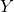-axis?
2. Does the graph have intersection points with the-axis, and if so, how many?
3. What is the symmetry axis?

### Solution

1. Because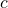in the formula equals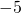, the intersection point with the-axis equals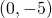.
2. In order to find out whether the graph of the function has intersection points with the-axis, we have to calculate the discriminant: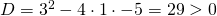The discriminant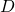is greater than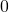and thus the graph has two intersection points with the-axis.

3. The symmetry axis is: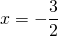0